Next: 4.8.4 Three-Dimensional Temperature Distribution Up: 4.8 Examples Previous: 4.8.2 An In-Segregation Model

The advantage of a flexible system is that even new approaches to problems that at first sight are not immediately connected with partial differential equations can be tested without great effort. One of these themes is the adaptation of a mesh respectively the reorganization of it in case of bad conditioned elements. The problem that is addressed to is as follows:

• Starting from a given mesh with a fixed connectivity structure a numerical model is searched to adjust the position of the nodes in a way that a minimum error of a given analytical criterion can be achieved. A new triangulation with the same topology but adapted to a given criterion is computed.

The presented method is a mixture of an adaptive local mesh refinement and coarsement using an error estimation and a moving mesh based on artificially introduced forces into an elasto-mechanical model depending on the geometry of the element. With AMIGOS as rapid prototyping system it was possible to reduce the rather complex elasto-mechanical differential equations used for relaxation to a very simple equation system that remains linear even in case of large displacements.

The first approach was, to optimize the angles of a single element by using linear elasticity and internal forces which are chosen to be proportional to the angles of the belonging element (Fig. 4.18).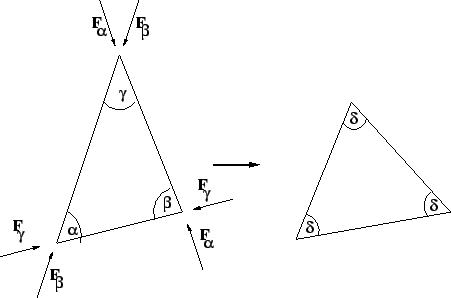For the elasto-mechanical equations the displacement vector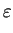=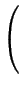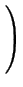=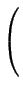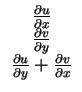=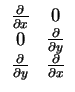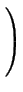.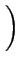(4.21)

has to be calculated in dependence of the stress tensor. In case of general linear elastic behavior, the relationship between stresses and strains are of the form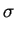= D . (-) +(4.22)

where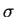is the calculated stress in dependence of the strain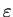andas well asare initial strains and stresses respectively.

The nodal forces were defined as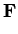=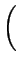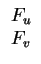(4.23)

with the components Fu and Fv corresponding to the directions of displacement.

The amount of forces is calculated in dependence of the angle of an element at node i

 Fi = k . (-)3 (4.24)

under the condition thatFi = 0 (4.25)

Unfortunately this attempt results in a nonlinear formulation depending on the actual state of the angles of a single element and therefore calculation time increases significantly. Furthermore, the parameter k of (4.24) is essential for convergence and can not be set to a satisfying general value suitable for any grid. Nevertheless, the first results were very promising and due to the dualism of mechanics (on the one hand high nodal stresses where displacement is not possible, e.g. boundary corners, and on the other hand total relaxation where the system has enough freedom to move) the expected effect of grid relaxation was clearly recognizable. To increase the freedom of the system even at the boundaries the only restriction required is to preserve the geometry. This can be achieved by moving boundary conditions as shown in Fig. 4.19.Due to the fact that the relaxation should be independent of the material, and shear stress is not the aim of interest the mechanical equations can be reduced drastically. All we are looking for is an energetic minimum of the whole system which is equivalent to the vanishing of all inner forces artificially imprinted into the system, just the geometry must be preserved. It turned out that this can be satisfied easily by forces compressing a single element minimizing the volume of each element. The forces have to be oriented along the edges with the condition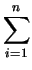Fi = 0 (4.26)

per element like in the first attempt. Because of pinned boundary conditions (geometry preservation) the triangles do not collapse. Due to the introduced forces each point moves towards its local optimum depending on the connectivity to other element points (Fig. 4.20). Furthermore the minimum volume does not depend on the mechanical behavior since the compression always tends towards zero volume. The resulting degenerated equation system can be written as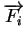=k .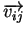(4.27)

whereare the forces at the element node i anddenotes the orientation of the force along the element edge from point i to point j.As a global result a stiffness matrix can be found where the total internal forces are minimized. The simplified model is linear and independent of the parameter k, since the optimum is always the same, no matter how strong the artificial compression forces are.

Unfortunately, there are some external influences to be considered. On the one hand, the boundary itself has less freedom in movement. This represents a massive bad influence on the optimum, which leads to the fact that sometimes a surface retriangulation might be necessary. But in most cases boundary conditions, where surface points are allowed to move along the edge, are sufficient to reach high quality grids (Fig. 4.19). On the other hand internal connectivities of elements can not be changed and therefore it sometimes leads to a deviation of the optimum (e.g. consider a point with a high number of connected elements - the optimum results in numerically bad triangles). A local retriangulation seems to be the only solution for this problem.

Nevertheless, since the influence mechanisms are quite simple, a mesh can also be extracted from an ortho-grid by splitting each rectangle into two triangles. As first example the interface between oxide and silicon of an oxidizing block is calculated using a level set method to distinguish between the two materials. The corresponding grid is adapted to the moving interface. During recalculation of the grid, using adaptive hierarchical split methods [Lei95] (Fig. 4.21), any concentration on the grid can be fitted and because of relaxation the resulting grid degradation is minimized by implicit smoothing (Fig. 4.22). The fact that a hierarchical adaptation which supports both, refinement and coarsement, is used is essential, otherwise the adaptation of a quantity would result in a miss-fitted adaptation due to grid movement.

The second example (Fig. 4.23) shows a similar calculation as in example one, but now the relaxation criterion is modified to adapt the interface with a changing orientation instead of a global relaxation. Thus the relaxation of rectangles smoothly changes from total relaxation to non-relaxation.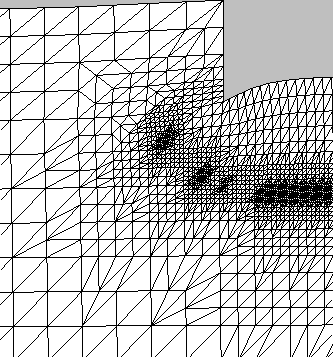The advantage of this method is, that it can immediately be used for three dimensions without any modifications. A simple a priori local adaptation allows to optimize local angles, which is essential in three dimensions. The third example (Fig. 4.24 and Fig. 4.25) shows an interface of oxygen and silicon adapted with the mesh relaxation method where the accuracy could be preserved with the advantage to reduce the number of mesh-points in comparison to non-relaxation methods.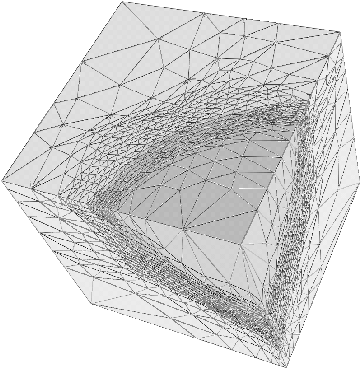Next: 4.8.4 Three-Dimensional Temperature Distribution Up: 4.8 Examples Previous: 4.8.2 An In-Segregation Model